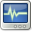Scilab Home page | Wiki | Bug tracker | Forge | Mailing list archives | ATOMS | File exchange
Change language to: English - Français - Português - 日本語 -

See the recommended documentation of this function

Справка Scilab >> Xcos > xcos

# xcos

Block diagram editor and GUI for the hybrid simulator

### Calling Sequence

```xcos()
xcos(filename)
xcos(scs_m_list)```

### Arguments

• filename : a character string containing the path of the diagram file (.cos, .cosf or .zcos extension). If no input argument is used, an empty diagram is opened (default name Untitled).

• scs_m_list :a Xcos diagram structure after edition.

### Description

Xcos is a graphical editor for constructing models of hybrid dynamical systems. Models can then be assembled, loaded, saved, compiled, simulated, using GUI of Xcos. Xcos serves as an interface to the various block diagram compilers and the hybrid simulator scicosim

The Xcos environment presents the following elements:

• Editor

The editor allows the design of flow charts representing a dynamic system made of blocks defined in palettes. Users can customize standard blocks and define new ones.

• Palette browser

The palette browser lists all Xcos standard blocks grouped by categories (signal processing, electrical, hydraulics, derivative, integral, etc.). See the figure below.

• Compiler/Simulator

Xcos environment enables, from the built model, the simulation of complex systems - discrete, continuous, defined by symbolic equations (Modelica), etc.. and hybrid systems combining the capabilities above. The data resulting of the simulation can then be graphically viewed in real time, used in Scilab for post processing...

Invoking xcos with the toolbar icon, with the Xcos menu item in Applications of the Scilab main window, or as the following example opens up the empty Xcos window of edition shown below.

`xcos`To open an existing diagram, you must provide a valid filename, like in this example:

`xcos("SCI/modules/xcos/demos/bounce.zcos")`

In this use case, the Xcos window is opened and displays the diagram ofbounce.zcos demonstration.

If a diagram structure is present in Scilab workspace, you can open it like in this example:

```loadXcosLibs();

// define diagram structure
scs_m = scicos_diagram();
scs_m.props.title = "Imported diagram";
scs_m.objs(1) = SUMMATION("define");
scs_m.objs(1).graphics.sz = [30 50];

xcos(scs_m);```

where scs_m is the diagram structure of bounce.zcos.

You build your models by linking the blocks provided in the palette browser simultaneously opened with Xcos editor. This window has the following appearanceYou can customize the palettes to be shown by clicking on the Palette title in the left part of the windows. The palette windows shows a selection list where you can select/deselect palettes individually. Tp return to the previous display, click on a palette title.Xcos may requires a C compiler for Modelica based models, for user defined blocks and code generation.

An installed supported compiler is recommended.

### Example

```xcos();
xcos(SCI+"/modules/xcos/demos/Discrete-KalmanFilter.zcos");
scs_m = scicos_diagram();
scs_m.objs(\$+1) = BIGSOM_f("define");
xcos(scs_m);```